Assertion : When a coil is joined to a cell, the current through the cell will be 10% less than its

steady state value at time , where $\mathrm{\tau }$ is the time constant of the circuit.

Reason : When a coil is joined to a cell, the growth of current is given by $\mathrm{i}={\mathrm{i}}_{0}{\mathrm{e}}^{-\mathrm{t}/\mathrm{\tau }}$

1. If both the assertion and the reason are true and the reason is a correct explanation of the assertion
2. If both the assertion and reason are true but the reason is not a correct explanation of the assertion
3. If the assertion is true but the reason is false
4. If both the assertion and reason are false

Concept Questions :-

LR circuit
High Yielding Test Series + Question Bank - NEET 2020

Difficulty Level:

Assertion: The magnetic flux linked with a coil is $\mathrm{\varphi }$, and the emf induced in it is $\mathrm{\epsilon }$. If $\mathrm{\varphi }=0$, $\mathrm{\epsilon }$ must be zero.

Reason :

(a) Assertion and Reason both are correct and the Reason is the correct explanation of the Assertion.

(b) Assertion and Reason both are correct but the Reason is not the correct explanation of the Assertion.

(c) The assertion is correct but the Reason is wrong.

(d) Assertion and Reason both are wrong.

1. If both the assertion and the reason are true and the reason is a correct explanation of the assertion
2. If both the assertion and reason are true but the reason is not a correct explanation of the assertion
3. If the assertion is true but the reason is false
4. If both the assertion and reason are false

Concept Questions :-

Faraday 's law and lenz law
High Yielding Test Series + Question Bank - NEET 2020

Difficulty Level:

Assertion : The coil in the resistance boxes are made by doubling the wire.

Reason : Thick wire is required in resistance box.

1. If both the assertion and the reason are true and the reason is a correct explanation of the assertion
2. If both the assertion and reason are true but the reason is not a correct explanation of the assertion
3. If the assertion is true but the reason is false
4. If both the assertion and reason are false

Concept Questions :-

Self-inductance
High Yielding Test Series + Question Bank - NEET 2020

Difficulty Level:

A coil of area  and of 50 turns is kept with its plane normal to a magnetic field B. A resistance of 30 ohm is connected to the resistance-less coil. B is 75 exp (– 200t) gauss. The current passing through the resistance at t = 5 ms will be-

1.

2.

3.

4.

Concept Questions :-

Faraday 's law and lenz law
High Yielding Test Series + Question Bank - NEET 2020

Difficulty Level:

A long solenoid having 1000 turns per cm is carrying alternating current of one ampere peak value. A search coil of area of cross-section  and of 20 turns is placed in the middle of the solenoid so that its plane is perpendicular to the axis of the solenoid. The search coil registers a peak voltage . The frequency of the current in the solenoid is -

1.  1.6 per second

2.  0.16 per second

3.  15.9 per second

4.  15.85 per second

Concept Questions :-

Faraday 's law and lenz law
High Yielding Test Series + Question Bank - NEET 2020

Difficulty Level:

Rate of increment of energy in an inductor with time in series LR circuit getting charge with battery of emf E is best represented by – [inductor has initially zero current]

1.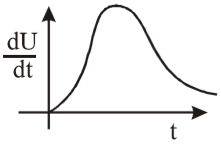2.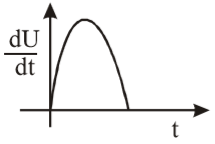3.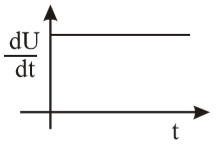4.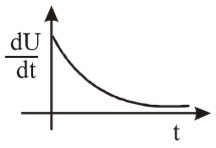Concept Questions :-

LR circuit
High Yielding Test Series + Question Bank - NEET 2020

Difficulty Level:

A copper rod of length 0.19 m is moving parallel to a long wire with a uniform velocity of 10 m/s. The long wire carries 5 ampere current and is perpendicular to the rod. The ends of the rod are at distances 0.01 m and 0.2 m from the wire. The emf induced in the rod will be-

1.

2.

3.

4.

Concept Questions :-

Motional emf
High Yielding Test Series + Question Bank - NEET 2020

Difficulty Level:

A conducting square loop of side L and resistance R moves in its plane with a uniform velocity v perpendicular to one of its sides.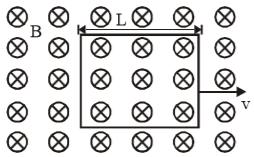A magnetic induction B, constant in time and space, pointing perpendicular and into the plane of the loop exists everywhere, see figure. The current induced in the loop is

1.  BLv/R clockwise

2.  BLv/R anticlockwise

3.  2 BLv/R anticlockwise

4.  zero

Concept Questions :-

Motional emf
High Yielding Test Series + Question Bank - NEET 2020

Difficulty Level:

A coil of radius 1 cm and  turns 100 is placed in the middle of a long solenoid of radius 5 cm and having 8 turns/cm. The mutual induction in millihenry will be-

1.  0.0316

2.  0.063

3.  0.105

4.  Zero

Concept Questions :-

Mutual-inductance
High Yielding Test Series + Question Bank - NEET 2020

Difficulty Level:

A circular coil of radius 5 cm has 500 turns of a wire. The approximate value of the coefficient of self induction of the coil will be -

1.

2.

3.

4.

Concept Questions :-

Self-inductance